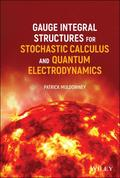John Wiley & Sons Gauge Integral Structures for Stochastic Calculus and Quantum ElectrodynamicsA stand-alone introduction to specific integration problems in the probabilistic theory of stochasti.. Product #: 978-1-119-59549-6 Regular price: \$107.48 \$107.48 Auf Lager

# Gauge Integral Structures for Stochastic Calculus and Quantum Electrodynamics1. Auflage Juni 2021
384 Seiten, Hardcover
Wiley & Sons Ltd

ISBN: 978-1-119-59549-6
John Wiley & Sons

Jetzt kaufen

### Preis: 115,00 €

ca.-Preis

Preis inkl. MwSt, zzgl. Versand

A stand-alone introduction to specific integration problems in the probabilistic theory of stochastic calculus

Picking up where his previous book, A Modern Theory of Random Variation, left off, Gauge Integral Structures for Stochastic Calculus and Quantum Electrodynamics introduces readers to particular problems of integration in the probability-like theory of quantum mechanics. Written as a motivational explanation of the key points of the underlying mathematical theory, and including ample illustrations of the calculus, this book relies heavily on the mathematical theory set out in the author's previous work. That said, this work stands alone and does not require a reading of A Modern Theory of Random Variation in order to be understandable.

Gauge Integral Structures for Stochastic Calculus and Quantum Electrodynamics takes a gradual, relaxed, and discursive approach to the subject in a successful attempt to engage the reader by exploring a narrower range of themes and problems. Organized around examples with accompanying introductions and explanations, the book covers topics such as:
* Stochastic calculus, including discussions of random variation, integration and probability, and stochastic processes.
* Field theory, including discussions of gauges for product spaces and quantum electrodynamics.
* Robust and thorough appendices, examples, illustrations, and introductions for each of the concepts discussed within.
* An introduction to basic gauge integral theory.

The methods employed in this book show, for instance, that it is no longer necessary to resort to unreliable "Black Box" theory in financial calculus; that full mathematical rigor can now be combined with clarity and simplicity. Perfect for students and academics with even a passing interest in the application of the gauge integral technique pioneered by R. Henstock and J. Kurzweil, Gauge Integral Structures for Stochastic Calculus and Quantum Electrodynamics is an illuminating and insightful exploration of the complex mathematical topics contained within.

I Stochastic Calculus 19

1 Stochastic Integration 21

2 Random Variation 33

2.1 What is Random Variation? 33

2.2 Probability and Riemann Sums 36

2.3 A Basic Stochastic Integral 38

2.4 Choosing a Sample Space 46

2.5 More on Basic Stochastic Integral 48

3 Integration and Probability 51

3.1 -Complete Integration 51

3.2 Burkill-complete Stochastic Integral 58

3.3 The Henstock Integral 59

3.4 Riemann Approach to Random Variation 63

3.5 Riemann Approach to Stochastic Integrals 66

4 Stochastic Processes 75

4.1 From R¯n to R¯ infinity 75

4.2 Sample Space R¯T with T Uncountable 83

4.3 Stochastic Integrals for Example 12 88

4.4 Example 12 93

4.5 Review of Integrability Issues 100

5 Brownian Motion 103

5.1 Introduction to Brownian Motion 103

5.2 Brownian Motion Preliminaries 110

5.3 Review of Brownian Probability 113

5.4 Brownian Stochastic Integration 116

5.5 Some Features of Brownian Motion 123

5.6 Varieties of Stochastic Integral 126

6 Stochastic Sums 135

6.1 Review of Random Variability 136

6.2 Riemann Sums for Stochastic Integrals 138

6.3 Stochastic Sum as Observable 141

6.4 Stochastic Sum as Random Variable 142

6.5 Introduction to integral T(dXs)² = t 145

6.6 Isometry Preliminaries 147

6.7 Isometry Property for Stochastic Sums 149

6.8 Other Stochastic Sums 153

6.9 Introduction to Itô's Formula 158

6.10 Itô's Formula for Stochastic Sums 160

6.11 Proof of Itô's Formula 161

6.12 Stochastic Sums or Stochastic Integrals? 163

II Field Theory 169

7 Gauges for Product Spaces 171

7.1 Introduction 171

7.2 Three-dimensional Brownian Motion 171

7.3 A Structured Cartesian Product Space 174

7.4 Gauges for Product Spaces 177

7.5 Gauges for Infinite-dimensional Spaces 180

7.6 Higher-dimensional Brownian Motion 187

7.7 Infinite Products of Infinite Products 192

8 Quantum Field Theory 199

8.1 Overview of Feynman Integrals 202

8.2 Path Integral for Particle Motion 206

8.3 Action Waves 208

8.4 Interpretation of Action Waves 211

8.5 Calculus of Variations 213

8.6 Integration Issues 217

8.7 Numerical Estimate of Path Integral 224

8.8 Free Particle in Three Dimensions 232

8.9 From Particle to Field 236

8.10 Simple Harmonic Oscillator 241

8.11 A Finite Number of Particles 247

8.12 Continuous Mass Field 253

9 Quantum Electrodynamics 261

9.1 Electromagnetic Field Interaction 261

9.2 Constructing the Field Interaction Integral 266

9.3 -Complete Integral Over Histories 269

9.4 Review of Point-Cell Structure 274

9.5 Calculating Integral Over Histories 275

9.6 Integration of a Step Function 279

9.7 Regular Partition Calculation 282

9.8 Integrand for Integral over Histories 284

9.9 Action Wave Amplitudes 287

9.10 Probability and Wave Functions 291

III Appendices 299

10 Appendix 1: Integration 303

10.1 Monstrous Functions 304

10.2 A Non-monstrous Function 305

10.3 Riemann-complete Integration 309

10.4 Convergence Criteria 314

10.5 "I would not care to y in that plane" 320

11 Appendix 2: Theorem 63 321

11.1 Fresnel's Integral 321

11.2 Theorem 188 of [MTRV] 326

11.3 Some Consequences of Theorem 63 Fallacy 331

12 Appendix 3: Option Pricing 333

12.1 American Options 333

12.2 Asian Options 340

13 Listings 353

13.1 Theorems 353

13.2 Examples 354

13.3 Definitions 356

13.4 Symbols 356